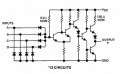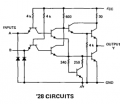# Voltage Drop from single resistor circuit (pull-up resistor)

#### abu1985

Joined Oct 18, 2015
74
I'm getting wrapped around the wheels here on some basic DC theory. I was looking up pull-up resistor applications, and while I understand that the resistor is needed to keep from shorting TTL to 0v, I'm confused as to how it sends proper voltage to the input pin.

This is more about voltage drop across resistors, and what voltage is on the "other side" of the resistor. In a voltage divider circuit, as I decrease the value of R1, the output voltage increases. This makes sense to me, because lowering the resistance of R1 is getting me "closer" to just a wire supplying the input voltage. But I've also read, that a low value resistor (10 ohm for example) will have a big voltage drop and not much voltage after the resistor.

Thinking about the pull-up resistor, a high ohm value is used (say 100k ohms) to draw a low amount of current at a logic 0 state, but also have a small voltage drop so the input pin of the IC will have pretty close to 5 volts at a logic 1 state?

I don't see how pull-up resistors work when I compare the theory to voltage divider circuits?

Also, I think I overthink the way voltage behaves in circuits. It's more important how the current behaves.

Any coaching is appreciated!

Have a good night (or day if you're out west)

#### dl324

Joined Mar 30, 2015
12,269
while I understand that the resistor is needed to keep from shorting TTL to 0v, I'm confused as to how it sends proper voltage to the input pin.
There's no issue with connecting a TTL input directly to ground unless you later want to be able to pull it up.

This is more about voltage drop across resistors, and what voltage is on the "other side" of the resistor. In a voltage divider circuit, as I decrease the value of R1, the output voltage increases. This makes sense to me, because lowering the resistance of R1 is getting me "closer" to just a wire supplying the input voltage. But I've also read, that a low value resistor (10 ohm for example) will have a big voltage drop and not much voltage after the resistor.
It would be helpful if you provided schematics for the scenarios you're discussing. When you use a pull-up or pull-down resistor, there's no voltage divider in the traditional sense.

On this gate, the inputs are diodes on the base of a transistor:On this gate, the inputs are emitters:Thinking about the pull-up resistor, a high ohm value is used (say 100k ohms) to draw a low amount of current at a logic 0 state, but also have a small voltage drop so the input pin of the IC will have pretty close to 5 volts at a logic 1 state?
If you're still talking about TTL, pull-ups would be closer to 1k.
I don't see how pull-up resistors work when I compare the theory to voltage divider circuits?
Post schematics for your specific questions so we know what you're referring to.

#### crutschow

Joined Mar 14, 2008
26,069
If you're still talking about TTL, pull-ups would be closer to 1k.
Why.
The pull-up resistor draws no current when the TTL input is high, so a higher value resistor can readily be used.

On the other hand, a pull-down TTL input resistor must be a low value, since it must sink the TTL input current while maintaining the voltage below the maximum logic-zero value.

#### MrChips

Joined Oct 2, 2009
22,574
There is only one law that you need to apply and that is Ohm's Law.

Here is Ohm's Law in its original form and its two corollaries:

I = V / R
R = V / I
V = I x R

Ignore a voltage divider circuit for a moment for this exercise.

What you need to know is that any node in a circuit, and in this case we are referring to the input pin of any logic gate, is a source or sink of current. Every logic family is different. Look up the data sheet of a typical 7400 gate as an example.

You will find two input current specs:
IIH = 40μA
IIL = -1.6mA

Let us look at what each of these two specs are saying.

1)
IIH = 40μA says that when the logic input is HIGH, the input pin sinks 40μA (current into the pin).
If Vcc = 5V and you want a guaranteed 4V on the logic input, then the pull-up resistor is

R = (5V - 4V) / 40μA = 25kΩ

2)
IIL = -1.6mA says that when the logic pin is LOW, 1.6mA (max) is flowing from the pin (hence the negative sign).
If you want a guaranteed 0.4V on the logic input, then the pull-down resistor is:

R = 0.4V / 1.6mA = 250Ω

Note that the specs for 74LS00 are different from that for 7400.

#### abu1985

Joined Oct 18, 2015
74
There is only one law that you need to apply and that is Ohm's Law.

Here is Ohm's Law in its original form and its two corollaries:

I = V / R
R = V / I
V = I x R

Ignore a voltage divider circuit for a moment for this exercise.

What you need to know is that any node in a circuit, and in this case we are referring to the input pin of any logic gate, is a source or sink of current. Every logic family is different. Look up the data sheet of a typical 7400 gate as an example.

You will find two input current specs:
IIH = 40μA
IIL = -1.6mA

Let us look at what each of these two specs are saying.

1)
IIH = 40μA says that when the logic input is HIGH, the input pin sinks 40μA (current into the pin).
If Vcc = 5V and you want a guaranteed 4V on the logic input, then the pull-up resistor is

R = (5V - 4V) / 40μA = 25kΩ

2)
IIL = -1.6mA says that when the logic pin is LOW, 1.6mA (max) is flowing from the pin (hence the negative sign).
If you want a guaranteed 0.4V on the logic input, then the pull-down resistor is:

R = 0.4V / 1.6mA = 250Ω

Note that the specs for 74LS00 are different from that for 7400.
Thanks for the reply. You have some detail here that made me think a little differently about it.

Take care